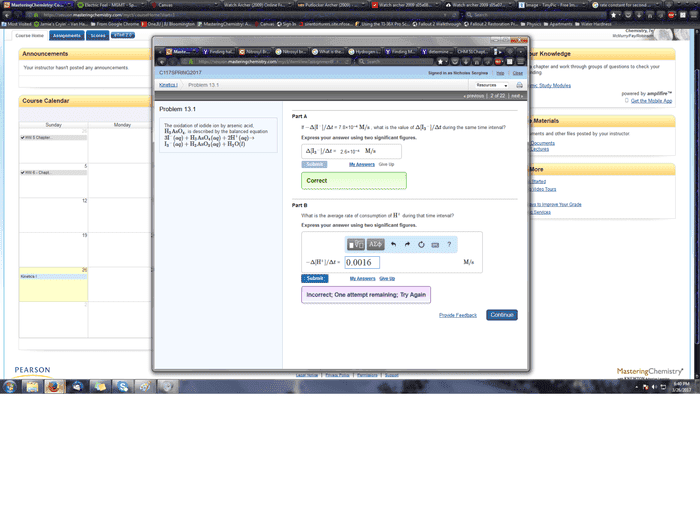# Average Reaction Rates

## Homework Statement## Homework Equations

Δ[A]/aΔt = Δ[ B]/bΔt

## The Attempt at a Solution

Δ[ I]/3Δt = Δ[H]/2Δt = 7.8E-4. What exactly am I supposed to do for this?

## Answers and Replies

mfb
Mentor
Δ/3Δt = Δ[H]/2Δt = 7.8E-4
I don't understand what you calculate here, and the number you get there (which is not the answer) doesn't agree with the number you put into the form (which is also not the answer).

Part B works in the same way as part A.

I don't understand what you calculate here, and the number you get there (which is not the answer) doesn't agree with the number you put into the form (which is also not the answer).

Part B works in the same way as part A.
What I did in part A was divide 7.8E-4 by 3. However I am unsure what this is fundamentally doing apparently as I can not understand how to get part B. Do I divide 7.8E-4 by 2?

mjc123
Science Advisor
Homework Helper
Δ[ I]/3Δt = Δ[H]/2Δt = 7.8E-4.
That is not true. -Δ[I-]/Δt = 7.8e-4 M/s (don't forget the units), as you were told. Given this, how would you apply Δ[ I-]/3Δt = Δ[H+]/2Δt to answer the question?

mfb
Mentor
You know ##I_3^-## is produced at 2.6*10-4 M/s. For every molecule of ##I_3^-## produced, two atoms of ##H^+## are used.
What is the rate of ##H^+## consumption?